Thursday , July 7 2022# NCERT 5th Class (CBSE) Mathematics: Fractions

### Dividing a Whole Number by a Fraction

(b) 3÷3/4 = ?

Think: How many 3/4 in 3?

Let us understand this with the help of an example.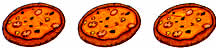Here are three pizzas.

How many 3/4 ths in three pizzas?

First cut each pizza into 1/4th pieces.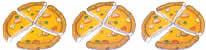Then separate them into 3/4ths.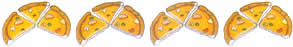Answer: There are four 3/4 ths in 3 pizzas.

Here is how you can solve this without pictures.

3÷3/4 = ?

Step 1. Find the reciprocal of the divisor. Reciprocal of 3/4 is 4/3

Step 2. Multiply the dividend with the reciprocal: 3×4/3 = 4

### Dividing a Fraction by a Whole Number

(c) Ajay has 3/4 l juice. If he pours it equally into 4 glasses, how much will each glass hold?

3/4÷4 = ?

3/4÷4 = 3/4÷4/1 = 3/4×i/4 = 3/16

Answer: Each glass will hold 3/16 l juice.

(d) 5/7÷1 = ?

5/7÷1/1 = 5/7×1/1 =5/7

5/7÷1 = 5/7

### Dividing a Fraction by a Fraction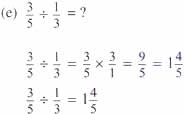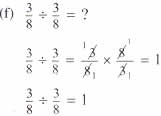## चुनौती हिमालय की 5th NCERT CBSE Hindi Rimjhim Ch 18

चुनौती हिमालय की 5th Class NCERT CBSE Hindi Book Rimjhim Chapter 18 प्रश्न: लद्दाख जम्मू-कश्मीर राज्य में …

### One comment

1.I need worksheet for Class 5th Mathematics.